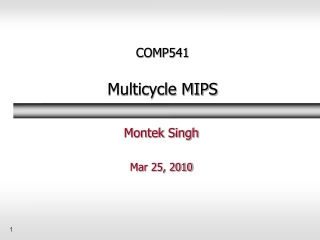DownloadDownload PresentationCOMP541 Multicycle MIPS

# COMP541 Multicycle MIPS

Download Presentation## COMP541 Multicycle MIPS

- - - - - - - - - - - - - - - - - - - - - - - - - - - E N D - - - - - - - - - - - - - - - - - - - - - - - - - - -
##### Presentation Transcript

1. COMP541Multicycle MIPS Montek Singh Mar 25, 2010

2. Topics • Issue w/ single cycle • Multicycle MIPS • State elements • Now add registers between stages • How to control • Performance

3. Multicycle MIPS Processor • Single-cycle microarchitecture: + simple • cycle time limited by longest instruction (lw) • two adders/ALUs and two memories • Multicyclemicroarchitecture: + higher clock speed + simpler instructions run faster + reuse expensive hardware on multiple cycles - sequencing overhead paid many times • Same design steps: datapath & control

4. Multicycle State Elements • Replace Instruction and Data memories with a single unified memory • More realistic

5. Multicycle Datapath: instruction fetch • First consider executing lw • STEP 1: Fetch instruction

7. Multicycle Datapath: lw immediate

9. Multicycle Datapath: lw memory read

10. Multicycle Datapath: lw write register

11. Multicycle Datapath: increment PC Now using main ALU when it’s not busy

12. Multicycle Datapath: sw • Already know how to generate addr • Write data in rt to memory

13. Multicycle Datapath: R-type Instrs. • Read from rs and rt • Write ALUResult to register file • Write to rd (instead of rt)

14. Multicycle Datapath: beq • Determine whether values in rs and rt are equal • Calculate branch target address: • BTA = (sign-extended immediate << 2) + (PC+4) • ALU reused

15. Complete Multicycle Processor

16. Control Unit

17. Main Controller FSM: Fetch

18. Main Controller FSM: Fetch • Fetch instruction • Also increment PC (because ALU not in use) Note: signals only shown when needed and enables only when asserted.

19. Main Controller FSM: Decode • No signals needed for decode • Register values also fetched • Perhaps will not be used

20. Main Controller FSM: Address Calculation • Now change states depending on instr

21. Main Controller FSM: Address Calculation • For lw or sw, need to compute addr

22. Main Controller FSM: lw • For lw now need to read from memory • Then write to register

23. Main Controller FSM: sw • sw just writes to memory • One step shorter

24. Main Controller FSM: R-Type • The r-type instructions have two steps: compute result in ALU and write to reg

25. Main Controller FSM: beq • beq needs to use ALU twice, so consumes two cycles • One to compute addr • Another to decide on eq • Can take advantage of decode when ALU not used to compute BTA • (no harm if BTA not used)

26. Complete Multicycle Controller FSM

27. Main Controller FSM: addi • Similar to r-type • Add • Write back

29. Extended Functionality: j

30. Control FSM: j

31. Control FSM: j

32. Multicycle Performance • Instructions take different number of cycles: • 3 cycles: beq, j • 4 cycles: R-Type, sw, addi • 5 cycles: lw • CPI is weighted average • SPECINT2000 benchmark: • 25% loads • 10% stores • 11% branches • 2% jumps • 52% R-type • Average CPI = (0.11 + 0.2)(3) + (0.52 + 0.10)(4) + (0.25)(5) = 4.12

33. Multicycle Performance • Multicycle critical path: • Tc = tpcq + tmux + max(tALU + tmux, tmem) + tsetup

34. Multicycle Performance Example Tc = tpcq_PC + tmux + max(tALU + tmux, tmem) + tsetup = tpcq_PC + tmux + tmem + tsetup = [30 + 25 + 250 + 20] ps = 325 ps

35. Multicycle Performance Example • For a program with 100 billion instructions executing on a multicycle MIPS processor • CPI = 4.12 • Tc = 325 ps • Execution Time = (# instructions) × CPI × Tc = (100 × 109)(4.12)(325 × 10-12) = 133.9 seconds • This is slower than the single-cycle processor (92.5 seconds). Why? • Not all steps the same length • Sequencing overhead for each step (tpcq + tsetup= 50 ps)

36. Review: Single-Cycle MIPS Processor

37. Review: Multicycle MIPS Processor

38. Next Time • We’ll look at pipelined MIPS • Adding throughput (and complexity) by trying to use all hardware every cycle